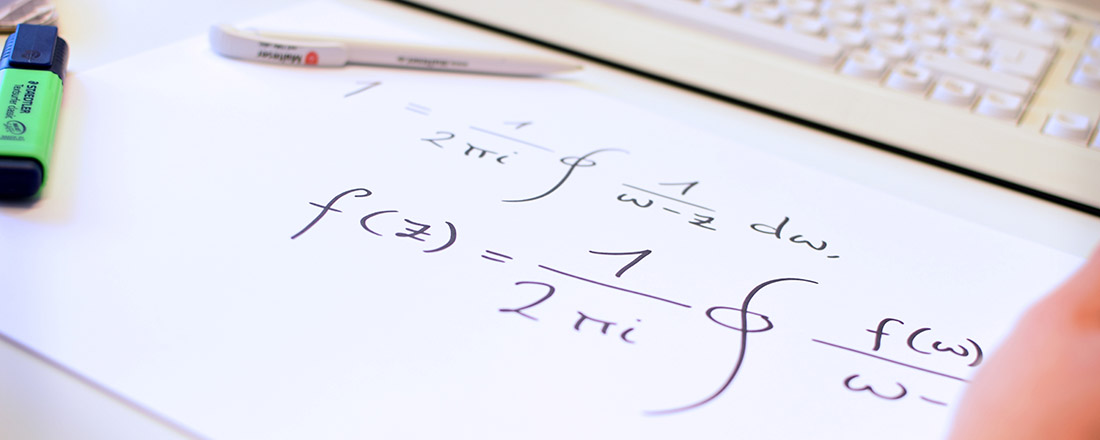## Faculty of Mathematics, Physics & Computer Science

### Chair of Mathematics I - Complex Analysis - Prof. Dr. Thomas Peternell

Print page# ​Welcome to the Chair of Complex Analysis

The chair deals with topics from complex geometry, i.e., the theory of complex varieties.

## Complex varieties occur in a wide variety of contexts

• as solution sets of polynomial equations (algebraic varieties)
• as spaces with certain curvature properties in differential geometric contexts
• as parameter spaces of geometric objects, such as curves, or
• as model spaces in physics, for example in quantum field theory (Calabi-Yau varieties)

## Many different areas of mathematics are used in the study of complex varieties:

• Real and complex analysis
• Differential geometry
• Commutative and homological algebra
• Topology